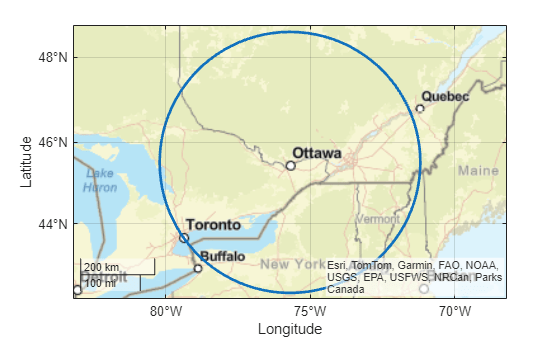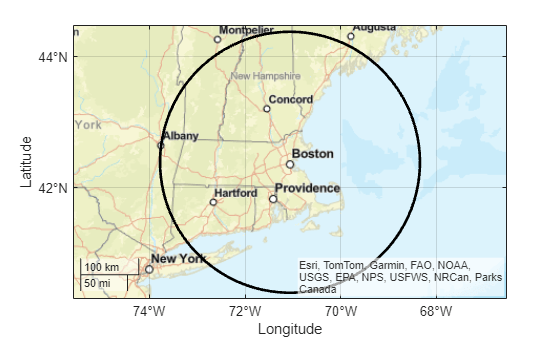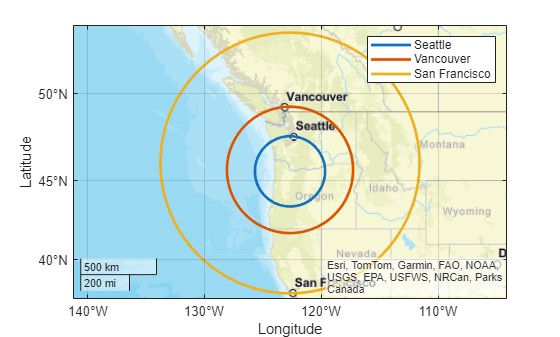# scircle2

Small circle from center and point on perimeter

## Syntax

``[lat,lon] = scircle2(lat0,lon0,latP,lonP)``
``[lat,lon] = scircle2(lat0,lon0,latP,lonP,ellipsoid)``
``[lat,lon] = scircle2(___,units)``
``[lat,lon] = scircle2(lat0,lon0,latP,lonP,ellipsoid,units,npts)``
``[lat,lon] = scircle2(method,___)``
``mat = scircle2(___)``

## Description

example

````[lat,lon] = scircle2(lat0,lon0,latP,lonP)` finds the latitude and longitude coordinates of a small circle. Specify the geographic coordinates of the center of the small circle using `lat0` and `lon0`. Specify a point on the perimeter of the small circle using `latP` and `lonP`. This syntax assumes that the coordinates are referenced to a sphere.```

example

````[lat,lon] = scircle2(lat0,lon0,latP,lonP,ellipsoid)` specifies the reference ellipsoid `ellipsoid` for the coordinates.```
````[lat,lon] = scircle2(___,units)` specifies the angle units `units` of the inputs and outputs, in addition to any combination of input arguments from the previous syntaxes.```
````[lat,lon] = scircle2(lat0,lon0,latP,lonP,ellipsoid,units,npts)` specifies the number of points `npts` to include in the small circle.```
````[lat,lon] = scircle2(method,___)`, where `method` is `"rh"`, specifies that distances from the center of the small circle are rhumb line distances. The default for `method` is `"gc"`, which specifies that distances from the center of the small circle are great circle distances (for a sphere) or geodesic distances (for an ellipsoid).```
````mat = scircle2(___)` returns the latitude and longitude coordinates of the small circle in the matrix `mat`.```

## Examples

collapse all

Find the latitude and longitude coordinates of a small circle centered on Ottawa with Toronto on the perimeter.

```lat0 = 45.4215; lon0 = -75.6972; latP = 43.6532; lonP = -79.3832; [lat,lon] = scircle2(lat0,lon0,latP,lonP);```

Plot the small circle on a map using a thick line.

```geoplot(lat,lon,"LineWidth",2) geobasemap streets```Create a World Geodetic System of 1984 (WGS84) reference ellipsoid with a length unit of kilometers.

`wgs84 = wgs84Ellipsoid("km");`

Find the latitude and longitude coordinates of a small circle centered on Boston with Albany at the perimeter.

```lat0 = 42.3601; lon0 = -71.0589; latP = 42.6526; lonP = -73.7562; [lat,lon] = scircle2(lat0,lon0,latP,lonP,wgs84);```

Plot the small circle on a map using a thick black line.

```geoplot(lat,lon,"k","LineWidth",2) geobasemap streets```You can use the `scircle2` function to find the coordinates of multiple small circles with the same center or with different centers.

Multiple Small Circles with the Same Center

Find the coordinates of three small circles centered on Portland, Oregon. Specify points on the perimeters of the circles using the coordinates of Seattle, Vancouver, and Calgary.

```lat0 = 45.5152; lon0 = -122.6784; latP = [47.6062 49.2827 37.7749]'; lonP = [-122.3321 -123.1207 -122.4194]'; cities = ["Seattle" "Vancouver" "San Francisco"]; [lat,lon] = scircle2(lat0,lon0,latP,lonP);```

Display the small circles on a map using thick lines. Differentiate the circles by including the perimeter cities in a legend.

```geobasemap streets hold on for i=1:size(lat,2) geoplot(lat(:,i),lon(:,i),"LineWidth",2,"DisplayName",cities(i)) end legend```Multiple Small Circles with the Different Centers

Find the coordinates of three small circles with different centers.

• The first small circle is centered on Boston and has New York City on its perimeter.

• The second small circle is centered on Washington, DC and has Philadelphia on its perimeter.

• The third small circle is centered on Chicago and has St. Louis on its perimeter.

```lat0 = [42.3601 38.9072 41.8781]'; lon0 = [-71.0589 -77.0369 -87.6298]'; latP = [40.7128 39.9526 38.627]'; lonP = [-74.0060 -75.1652 -90.1994]'; [lat,lon] = scircle2(lat0,lon0,latP,lonP); cities = ["Boston and New York City" "Washington, DC and Philadelphia" "Chicago and St. Louis"];```

Display the small circles on a map using thick lines. Differentiate the circles by including the city name pairs in a legend.

```figure geobasemap streets hold on for i=1:size(lat,2) geoplot(lat(:,i),lon(:,i),"LineWidth",2,"DisplayName",cities(i)) end legend```## Input Arguments

collapse all

Latitude of the center of the small circle, specified as a scalar or a column vector.

• To find the coordinates of multiple small circles with the same center, specify `lat0` and `lon0` as scalars and specify `latP` and `lonP` as column vectors. The number of elements in the column vectors is the number of small circles.

• To find the coordinates of multiple small circles with different centers, specify `lat0`, `lon0`, and `latP` and `lonP` as column vectors of the same size.

The sizes of `lat0` and `lon0` must match.

Data Types: `double`

Longitude of the center of the small circle, specified as a scalar or a column vector.

• To find the coordinates of multiple small circles with the same center, specify `lat0` and `lon0` as scalars and specify `latP` and `lonP` as column vectors. The number of elements in the column vectors is the number of small circles.

• To find the coordinates of multiple small circles with different centers, specify `lat0`, `lon0`, and `latP` and `lonP` as column vectors of the same size.

The sizes of `lat0` and `lon0` must match.

Data Types: `double`

Latitude of a point on the perimeter of the small circle, specified as a scalar or a column vector.

When `lat0` and `lon0` are column vectors, the number of elements in `latP` must match the number of elements in `lat0` and `lon0`.

The sizes of `latP` and `lonP` must match.

Data Types: `double`

Longitude of a point on the perimeter of the small circle, specified as a scalar or a column vector.

When `lat0` and `lon0` are column vectors, the number of elements in `lonP` must match the number of elements in `lat0` and `lon0`.

The sizes of `latP` and `lonP` must match.

Data Types: `double`

Reference ellipsoid, specified as a `referenceSphere` object, a `referenceEllipsoid` object, an `oblateSpheroid` object, or a two-element numeric vector of the form `[semimajor_axis eccentricity]`, where `semimajor_axis` is the length of the semimajor axis and `eccentricity` is the eccentricity. The values `semimajor_axis` and `eccentricity` must be of data type `double`.

The default value of `[1 0]` represents the unit sphere.

Angle unit, specified as one of these options:

• `"degrees"` — Degrees

• `"radians"` — Radians

Data Types: `char` | `string`

Number of points to include in the small circle, specified as a scalar. If you specify more than one small circle, then the function finds `npts` points per small circle.

Data Types: `double`

Type of distance from the center of the small circle, specified as one of these options:

• `"gc"` — Use the great circle distance.

• `"rh"` — Use the rhumb line distance.

Data Types: `char` | `string`

## Output Arguments

collapse all

Latitude coordinates of the small circle, returned as a column vector with `npts` elements or a matrix of size `npts`-by-m, where m is the number of small circles.

Longitude coordinates of the small circle, returned as a column vector with `npts` elements or a matrix of size `npts`-by-m, where m is the number of small circles.

Latitude and longitude coordinates of the small circle, returned as a matrix equivalent to `[lat lon]`.

collapse all

### Small Circle

A small circle is the set of points with the same surface distance from a given center. Examples of small circles include the set of points exactly 100 miles from the Washington Monument and parallels on the globe.

## Version History

Introduced before R2006a